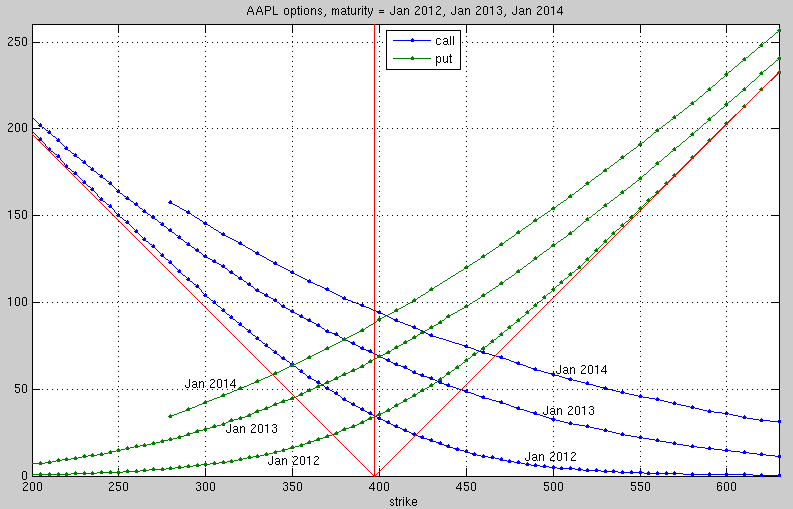NEWS:
• Final Exam
You can bring a cheat sheet: letter size, 2-sided, must be handwritten
topics for final and problems
solutions
• Exam 2 was on Monday, Dec. 5.
Solution
corrected version: Topics and practice problems ,   solutions
• Matlab m-files:
• Practice problems for Exam 1
solution
• Exam 1 is on Mon, Oct. 17
Solution of Exam
It covers the following topics:
• Present Value Analysis (Section 2 in the notes)
write Matlab code to find answer (payment or interest rate)
You may need fzero command.
• Probability: expectation, variance, conditional expectation (Sections 3.1-3.10)
• No Arbitrage Principle, Comparison principle (Sections 4.6)
• Option pricing with risk-neutral measure (Sections 4.9,4.10)
• Binomial tree model, trinomial tree model (Sections 4.1,4.2)
• Option pricing algorithm for binomial tree model, Delta hedging (Sections 4.11, Conclusion on p.36, 4.17-4.20)
• Properties of option prices (Section 5)
• Assignment 2 is due on Wed, Oct. 12, 9pm
Solution of problem 1, m-file a2p1ab.m
Solution of problem 2, m-files: xbinom_eu_put.m, xbinom_am_put.m, xbinom_am_call.m
Hand in printouts of all m-files you used (unless they are exactly the m-files which I provided). Use publish in Matlab. I need to see all code and the generated output and graphics.
• Option pricing with binomial trees in Matlab (see course notes):
binom_eu.m, binom_am.m, xbinom_eu_call.m
• Download the m-file xbinom_eu_call.m. Then run the example from section 4.19 in the class notes:
S0=10; K=11; [V,av] = xbinom_eu_call(.01,S0,1.1,.9,10,K,3);
• Course notes (Nov. 2), Appendix: Convex separation theorem
• Solution of Assignment 1, m-file Please look at this solution and make sure that you understand the Matlab code.
In particular look at the Matlab code for "Problem 3(a), Method 2" where I use vectors in Matlab. You will need this for future assignments.
• Assignment 1, due Wed Sept. 14 at 9pm
• Use the links below to find the price for an Apple (AAPL) put option and call option with strike price \$100 and maturity June 2017.
• If you are not familiar with Matlab do the following NOW:
• Read the "gentle introduction to Matlab" below
• Read the first 10 pages of the Matlab Primer (see below) AND TRY OUT THE COMMANDS ON A COMPUTER.
• Figure out how to write, run, and publish an m-file.AAPL option prices in Sept. 2011 for three different maturities

## Matlab programming

We will use Matlab for all computations.